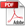# Search Developer's Guide — Chapter 12

## Using Aggregate Functions

This chapter describes how to use builtin aggregate functions and aggregate user-defined functions (UDFs) to analyze values in lexicons and range indexes.

This chapter contains the following sections:

### Introduction to Aggregate Functions

An aggregate function performs an operation over the values in one or more range indexes. For example, computing a sum or count over an element, attribute, or field range index. Aggregate functions are best used for analytics that produce a small number of results, such as computing a single numeric value across a set of range index values.

Aggregate functions use In-Database MapReduce, which greatly improves performance because:

• Analysis is parallelized across the hosts in a cluster, as well as across the database forests on each host.
• Analysis is performed close to the data.

MarkLogic Server provides builtin aggregate functions for common mathematical and statistical operations. You can also implement your own aggregate functions, using the Aggregate UDF interface. For details, see Implementing an Aggregate User-Defined Function in the Application Developer's Guide.

### Using Builtin Aggregate Functions

MarkLogic Server provides the following builtin aggregate functions, accessible through the XQuery, REST, and Java APIs.

XQuery Function REST and Java Aggregate Name
`cts:avg-aggregate` `avg`
`cts:correlation` `correlation`
`cts:count-aggregate` `count`
`cts:covariance` `covariance`
`cts:covariance-p` `covariance-population`
`cts:max` `max`
`cts:median` `median`
`cts:min` `min`
`cts:stddev` `stddev`
`cts:stddev-p` `stddev-population`
`cts:sum-aggregate` `sum`
`cts:variance` `variance`
`cts:variance-p` `variance-population`

The table below summarizes how to call an aggregate function directly using the XQuery, REST and Java APIs:

Interface Mechanism Example
XQuery Call the builtin function directly from your XQuery code.
```cts:sum-aggregate```(
cts:element-reference(
xs:QName("Amount")
)
)``````
REST Send a GET `/`version`/values/`{name} request, naming the function in the `aggregates` request parameter. For details, see Analyzing Lexicons and Range Indexes With Aggregate Functions in the REST Application Developer's Guide.
``````GET
/v1/values/amount?options=my-index-defns&```aggregate=sum```
Java Specify the aggregate name using `ValuesDefinition.setAggregate` and pass the `ValuesDefinition` to `QueryManager.values` or `QueryManager.tuples`. For details, see Java Application Developer's Guide.
```QueryManager qm = ...;
ValuesDefinition vdef =
qm.newValuesDefinition(...);
vdef.setAggregate("sum");```
```TuplesHandle t =
qm.tuples(vdef,
new TuplesHandle());```
Node.js Specify the aggregate name using `valuesBuilder.aggregates` and use `DatabaseClient.values.read` to perform the computation. For details, see Analyzing Lexicons and Range Indexes with Aggregate Functions in the Node.js Application Developer's Guide.
```const vb = marklogic.valuesBuilder;

vb.fromIndexes('Amount')
.aggregates('sum')
.slice(0)
)...```

You can also specify an aggregate function in a `<values/>` or `<tuples/>` element of query options. For example:

```<options xmlns="http://marklogic.com/appservices/search">
<values name="my-values">
<aggregate apply="sum" />
...
</values>
</options>```

For more details, see Search API: Understanding and Using, the Java Application Developer's Guide, the REST Application Developer's Guide, or the Node.js Application Developer's Guide.

### Using Aggregate User-Defined Functions

You can create an aggregate user-defined function (UDF) to analyze the values in one or more range indexes. An aggregate UDF must be installed before you can use it. For information on creating and installing aggregate UDFs, see Aggregate User-Defined Functions in the Application Developer's Guide.

Aggregate UDFs are best for analyses that compute a small number of results over the values in one or more range indexes, rather than analyses that produce results in proportion to the number of range index values or the number of documents processed.

UDFs are identified by a relative path and a function name. The path is the path under which the plugin is installed. The path is scope`/`plugin-id, where scope is the scope passed to plugin:install-from-zip when the plugin is installed, and plugin-id is the ID specified in `<id/>` in the plugin manifest. For details, see Installing a Native Plugin in the Application Developer's Guide.

The following example uses an aggregate UDF called myAvg that is provided by the plugin installed with the path `native/sampleplugin`:

`cts:aggregate("native/sampleplugin", "myAvg", ...)`

The table below summarizes how to invoke aggregate UDFs in XQuery, Java, and RESTful applications.

You can only pass extra parameters to an aggregate UDF from XQuery.

Interface Mechanism Example
XQuery Call `cts:aggregate`, supplying the path to the native plugin that implements the aggregate and the aggregate name. Pass aggregate-specific parameters through the 4th argument.
``````cts:aggregate(
```  "native/samplePlugin",
"myAvg",
```  cts:element-reference(
xs:QName("Amount")),
(plugin-arg1, plugin-arg2)
)``````
REST Send a GET request to the `/values/{name}` service, supplying the path to the native plugin in `aggregatePath` and the function name in `aggregate`. For details, Analyzing Lexicons and Range Indexes With Aggregate Functions in the REST Application Developer's Guide.
``GET /v1/values/amount?options=myoptions&`aggregatePath=native/samplePlugin&aggregate=myAvg`
Java Set the aggregate name and path on a `ValuesDefinition`, then pass the `ValuesDefinition` to `QueryManager.values` or `QueryManager.tuples`. For details, see the Java Application Developer's Guide.
```QueryManager qm = ...;
ValuesDefinition vdef =
qm.newValuesDefinition(...);
vdef.setAggregate("myAvg");
vdef.setAggregatePath(
"native/samplePlugin");```
```TuplesHandle t =
qm.values(vdef,
new ValuesHandle());```
Node.js Set the aggregate name and path using `valuesBuilder.udf` and `valuesBuilder.aggregates,` then use `DatabaseClient.values.read` to perform the computation. For details, see Analyzing Lexicons and Range Indexes with Aggregate Functions in the Node.js Application Developer's Guide.
```const vb = marklogic.valuesBuilder;

vb.fromIndexes('Amount')
.aggregates(
vb.udf(
'/native/samplePlugin,
'myAvg'))
.slice(0)
)...```

You can also specify an aggregate UDF in a `<values/>` or `<tuples/>` element of query options. For example:

```xquery version "1.0-ml";
import module namespace search = "http://marklogic.com/appservices/search"
at "/MarkLogic/appservices/search/search.xqy";

<options xmlns="http://marklogic.com/appservices/search">
<values name="my-values">
<aggregate apply="myAvg" udf="native/samplePlugin" />
...
</values>
</options```

For more details, see Search API: Understanding and Using, the Java Application Developer's Guide, or the REST Application Developer's Guide.

« Previous chapter
Next chapter »